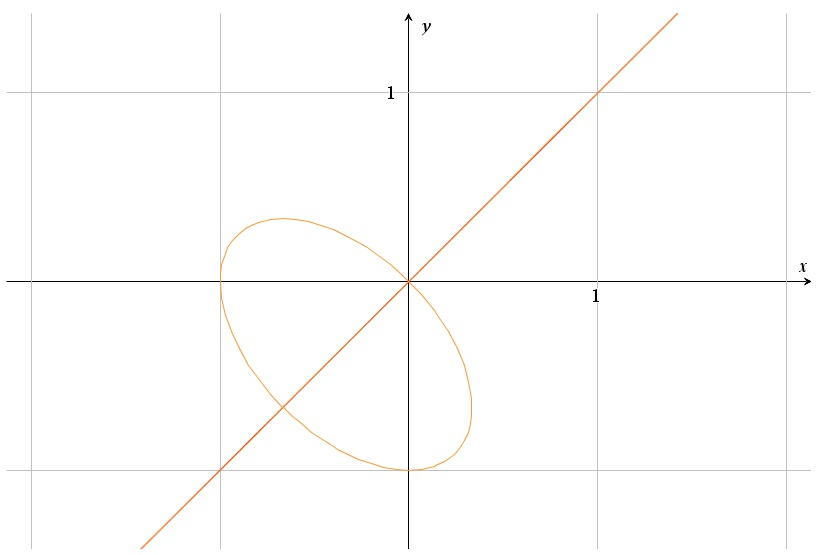# The relation y^3+y^2=x^3+x^2

Up a level : Differentiation, derivatives
Previous page : The circle and implicit derivatives
Next page : y^3+y^3=x^2+xLet us say we should graph${y^3} + {y^2} = {x^3} + {x^2}$

Technically we could make y the subject of it, but the solution is horrid (try on say Mathematica, Wolfram Alpha or TI Nspire CAS and you will see) and not very useful, so let us try to analyse it instead. To start with we can see that all points such that x=y are on the graph.

We can also see that x and y are used in a totally symmetric way, so we now that if a point (a, b) is on the graph then the point (b, a) is on the graph too.

Zeroes

Next we look for x and y intercepts.  If x=0 we get${y^3} + {y^2} = 0$

Or${y^2}(y + 1) = 0$

So y=0 or y=–1. If y=0 we get that$0 = {x^3} + {x^2}$

This gives x=0 or x= –1.

For y=–1 we get${( - 1)^3} + {1^2} = 0 = {x^3} + {x^2}$

That we have already dealt with. So we now have a line y=x and two points (0, –1) and (–1, 0)

Critical points – Horizontal tangents

To find a point with a horizontal tangent we should find points where
y´=0.

We thus need to find the implicit derivative of our relation. We get$3{y^3}y' + 2yy' = 3{x^2} + 2x$

or$y'(3{y^3} + 2y) = 3{x^2} + 2x$

So if y´=0 we get$0 = 3{x^2} + 2x = x(3x + 2)$

And thus x=0 or x=–2/3. Next we can find the y-values by substituting in these values in the original equation For  x=0 we have already found y=0 and y=–1.

The solution (0, 0) does not work since that is on the already established line y=x, that clearly does not have the slope 0.  And if you let x=0 and y=0 in the equation for the derivative that we can get from the result we got after the implicit derivative we get that y´=0/0, i.e. undefined. So what could happen at that point? Asking my student this one student replied after a short moment of thought that the graph might cross itself there, which indeed is the case. Nota bene that this was my introductory problem for implicit derivative.

So we have a point with the slope 0 at (0 ,1).

For x=−2/3 we get$\begin{gathered} {y^3} + {y^2} = {\left( { - \frac{2}{3}} \right)^3} + {\left( { - \frac{2}{3}} \right)^2} = - \frac{8}{{27}} + \frac{4}{9} \hfill \\ \quad \quad \quad = - \frac{8}{{27}} + \frac{{12}}{{27}} = \frac{4}{{27}} \hfill \\ \end{gathered}$

So we need to solve the equation${y^3} + {y^2} - \frac{4}{{27}} = 0$

Since we have x=–2/3, by symmetry we must have have y=–2/3 as one root. With a bit of algebra we can find that  y=1/3 is another solution – but that is not what we should concentrate on now, so, say we have just fond the roots in some way.*

We can discard the solution y=−2/3 since it is on the y=x line. And as before we can suspect that the graph cross itself at that point.  That leaves us with the point (−2/3,  1/3).

Vertical tangents

Of symmetry reasons these must be at (1, 0) and (1/3,  −2/3). This gives us:How to connect these points in some reasonable way? It looks like it could be some kind of ellipse there, and indeed, if we graph it we get:Rather cool I must say.  Next thing is to prove that the ellipse-like figure actually is an ellipse, but I leave that to you to figure out, at least for the moment.

* We may, for example, rewrite the equation as$27{{y}^{3}}+27{{y}^{2}}-4=0$

We can then rewrite this as${{\left( {3y} \right)}^{3}}+3{{\left( {3y} \right)}^{2}}-4=0$

or, after the substitution 3y=w.${{w}^{3}}+3{{w}^{2}}-4=0$

We can easily see that w=1 is a solution to this, and from y=–2/3, that we already know, we can see that w=–2 is another root. This is by the way a double root.

We thus get that y=1/3, and the other solution, y=–2/3, we already know.Up a level : Differentiation, derivatives
Previous page : The circle and implicit derivatives
Next page : y^3+y^3=x^2+xLast modified: Nov 2, 2023 @ 20:13